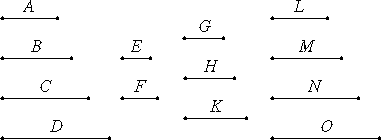# Proposition 3

If as many numbers as we please in continued proportion are the least of those which have the same ratio with them, then the extremes of them are relatively prime.

Let as many numbers as we please, A, B, C, and D, in continued proportion be the least of those which have the same ratio with them.

I say that the extremes of them, A and D, are relatively prime.Take two numbers E and F, the least that are in the ratio of A, B, C and D, then three others G, H and K with the same property, and others, more by one continually, until the multitude taken becomes equal to the multitude of the numbers A, B, C, and D. Let them be L, M, N, and O.

Since E and F are the least of those which have the same ratio with them, therefore they are relatively prime. And, since the numbers E and F multiplied by themselves respectively make the numbers G and K, and multiplied by the numbers G and K respectively make the numbers L and O, therefore both G and K and L and O are relatively prime.

And, since A, B, C, and D are the least of those which have the same ratio with them, while L, M, N, and O are the least that are in the same ratio with A, B, C, and D, and the multitude of the numbers A, B, C, and D equals the multitude of the numbers L, M, N, and O, therefore the numbers A, B, C, and D equal the numbers L, M, N, and O respectively. Therefore A equals L, and D equals O.

And L and O are relatively prime. Therefore A and D are also relatively prime.

Therefore, if as many numbers as we please in continued proportion are the least of those which have the same ratio with them, then the extremes of them are relatively prime.

Q.E.D.

## Guide

This proposition, the converse of VIII.1, says if a continued proportion with constant ratio is in lowest terms, then its end numbers are relatively prime.

The proof begins by using the previous proposition VIII.2 to construct the continued proportion in lowest terms, which must be the same as the given continued proportion, and it has relatively prime end numbers.

#### Use of this proposition

This proposition is used in propositions VIII.6, VIII.8, and VIII.21.